# Mathematical induction

Mathematical induction is a technique for proving a proposition that can be considered a conjunction of an infinite sequence of special cases. Despite the name, it is a deductive, rather than inductive, method, in the sense in which those terms are usually used in logic.

In any proof by mathematical induction, one proves that each member of an infinite sequence of propositions holds by reasoning as follows:

• The first case is true; and
• If any case is true then so is the next.

Suppose one calls the propositions P1, P2, P3, ... . Then the proof consists of two steps:

• The proof that P1 is true; and
• The proof that if Pk is true, the so is Pk+1, regardless of the value of k.

The first step is called the "basis". The second is the "inductive step". In the inductive step one assumes the truth of Pk and proves Pk+1. The proposition Pk is called the "induction hypothesis".

## Examples

### An identity

A proposition states that for all positive integers n,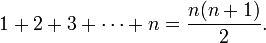$1 + 2 + 3 + \cdots + n = \frac{n(n+1)}{2}.$

In case n = 1, this says$1 = \frac{1(1 + 1)}{2},$

which is trivial. The induction hypothesis states that for a particular value of n we have$1 + 2 + 3 + \cdots + n = \frac{n(n+1)}{2},$

and in the induction step we need to prove that if the induction hypothesis is true, then so is the next proposition in the sequence, involving n + 1 instead of n: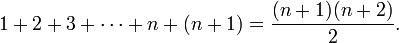$1 + 2 + 3 + \cdots + n + (n + 1) = \frac{(n+1)(n+2)}{2}.$

We have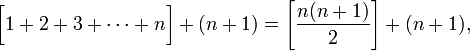$\bigg[ 1 + 2 + 3 + \cdots + n \bigg] + (n + 1) = \left[\frac{n(n + 1)}{2}\right] + (n + 1),$

since the induction hypothesis tells us the two expressions in square brackets are equal. Then by elementary algebra we have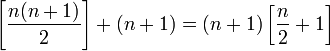$\left[\frac{n(n + 1)}{2}\right] + (n + 1) = (n+1)\left[ \frac{n}{2} + 1 \right]$

(we have taken out the factor n + 1 that the two terms share in common)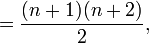${} = \frac{(n + 1)(n + 2)}{2},$

which was to be proved.

### An example from graph theory

Proposition: A tree in graph theory has Euler characteristic −1.

Proof: By induction,

Base proposition: the trivial tree Γ0—a single vertex without edges—has Euler characteristic Χ(Γ0) = 0E − 1V = − 1.

Induction step: For a tree Γ, and any extension single vertex extension of that tree Γ', show that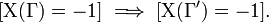$[ \Chi(\Gamma) = -1 ] \implies [ \Chi(\Gamma') = -1 ].$

If Χ(Γ) = − 1, then adding one vertex and one edge to this graph would yield:

Χ(Γ') = Χ(Γ) + 1E − 1V = Χ(Γ) = − 1.

Since all trees can be constructed in this manner from the trivial tree, it must be the case that all trees have Euler characteristic −1. QED.Some content on this page may previously have appeared on Citizendium.